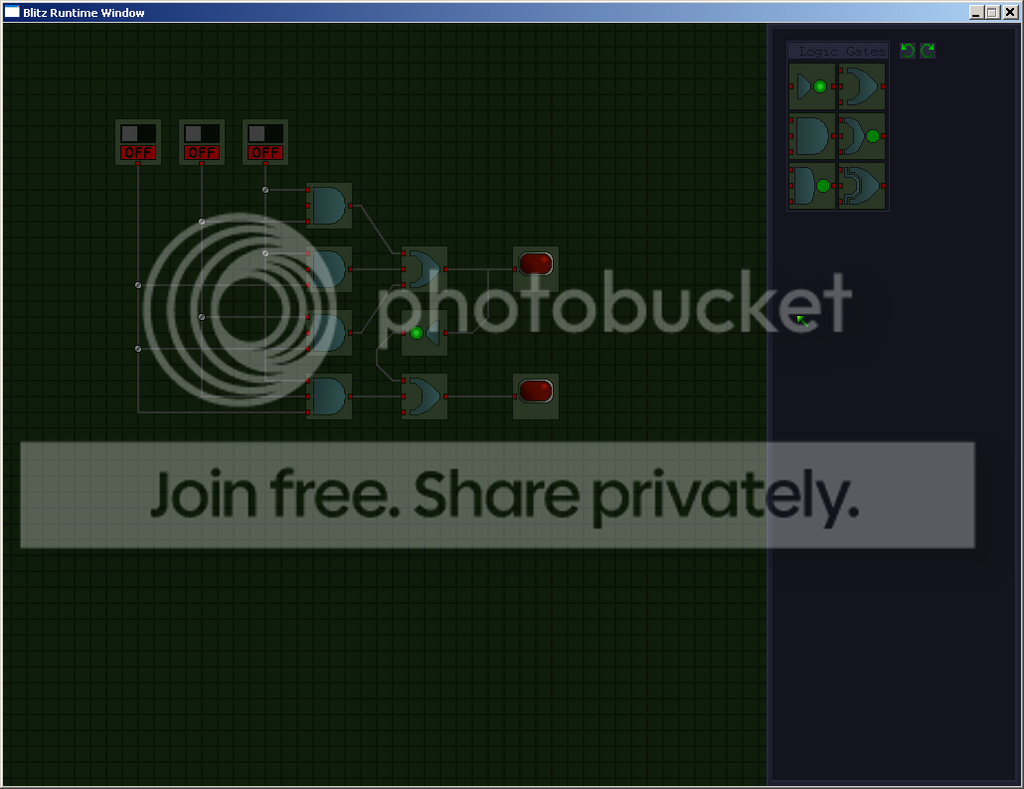-=+=- -=+=- -=+=- -=+=- -=+=- -=+=- -=+=- -=+=- -=+=- -=+=- -=+=- -=+=- -=+=- -=+=- -=+=- -=+=- -=+=- -=+=- -=+=- -=+=- -=+=- -=+=- -=+=- -=+=- -=+=- -=+=- -=+=- -=+=- -=+=- -=+=- (c) WidthPadding Industries 1987 0|81|0 -=+=- -=+=- -=+=- -=+=- -=+=- -=+=- -=+=- -=+=- -=+=- -=+=- -=+=- -=+=- -=+=- -=+=- -=+=- -=+=- -=+=- -=+=- -=+=- -=+=- -=+=- -=+=- -=+=- -=+=- -=+=- -=+=- -=+=- -=+=- -=+=- -=+=-
SoCoder -> Blogs Home -> Blogs

mike_gCreated : 08 November 2006
Edited : 08 November 2006

### Logic Boxthis is just a screenie of a little puzzle game I started making last night.

Basically you will try to make curcuits that do different things, like the one I made above, using as little components and wire as possible.

Anyway I hope i'll get round to finishing this. Might even whack in some cristmas pud or something else tacky, & christmasy so I can enter it for the dev-crunch compo.Looks like a fun game. Gotta love puzzle games!Looks good, you might wanna scale down the screenshot a little, tho